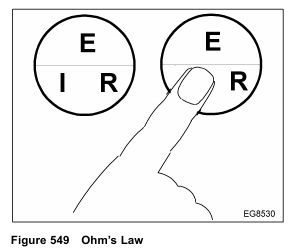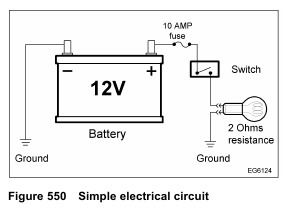﻿ International DT466 – DIAGNOSTIC TOOLS AND ACCESSORIES – Electronic Circuit Testing – Ohm’s Law – Diesel Engines Troubleshooting

## International DT466 – DIAGNOSTIC TOOLS AND ACCESSORIES – Electronic Circuit Testing – Ohm’s Law

Ohm’s Law describes the relationship between current, voltage, and resistance in an electrical circuit. Ohm’s Law also provides the basic formula for calculations.Memorize the formula in the circle. Cover the letter with a finger for the desired formula. For example, I is
covered, the formula is I = E ÷ R.

If two values are known for a given circuit, the missing one can be found by substituting the values in amperes, volts, or ohms.
The three basic formulas for Ohm’s Law are as follows:
I = Current (amperes)
E = Voltage (volts)
R = Resistance (ohms)

• I=E÷R
This formula states that the current flow (I) in the circuit equals the voltage (E) applied to the circuit divided by the total resistance (R) in the circuit. This shows that an increase in voltage or a decrease in resistance increases the current flow.
• E=I×R
This formula states that the voltage (E) applied to the circuit equals the current flow (I) in the circuit multiplied by the total resistance (R) in the circuit.
The voltage drop is caused by resistance across a particular load device in a series of load devices.
• R=E÷l
This formula states that the total resistance (R) in the circuit equals the voltage (E) applied to the circuit divided by the current flow (I) in the circuit.
Resistance can be calculated for a specific current flow when a specific voltage is applied.In a typical circuit, battery voltage is applied to a bulb through a 10 amp fuse and a switch. Closing the
switch turns on the bulb.
To find the current flow, use the formula I = E ÷ R:

Fill in the numbers for the formula:
I = 12 V ÷ 2 ohms
I = 6 amps
The bulb in this circuit operates at 6 amps and is rated at 6 amps. With 12 volts applied, the bulb will glow at
the rated output level (candlepower rating). However,
• If the voltage applied is low (low battery), the value of E is lower, current flow will be less, and the bulb
will glow less brightly.
• If connections are loose or the switch is corroded, the circuit resistance will be greater (value of R will be larger),         the current flow will be reduced, and the bulb will glow less brightly.

Voltage drops are important for the following reasons:
• High voltage drops indicate excessive resistance. For example, if a blower motor runs too slowly or a light glows           too dimly, the circuit may have excessive resistance. Voltage drop readings can isolate problems in parts of a                 circuit (corroded or loose terminals, for example).
• Too low of a voltage drop indicates low resistance. For example, if a blower motor runs too fast, the problem could       be low resistance in a resistor pack.
• Maximum allowable voltage drop under load is critical, especially for more than one high resistance problem.
All voltage drops in a circuit are cumulative. Corroded terminals, loose connections, damaged wires or other                similar conditions create undesirable voltage drops that decrease the voltage available across the key components      in the circuit. Increased resistance will decrease current flow in the circuit, preventing other components from            operating at peak efficiency. A small drop across wires (conductors), connectors, switches, etc., is normal because        all conductors have some resistance, but the total should be less than 10% of the total voltage drop in the circuit.

Using the Digital Multimeter
The following electrical test equipment should be available for testing electronic circuits:
• Voltmeter
• Ohmmeter

• Ammeter
• Jumper wires
• Test lights

24. November 2021 by samuel
Categories: International DT466 | Comments Off on International DT466 – DIAGNOSTIC TOOLS AND ACCESSORIES – Electronic Circuit Testing – Ohm’s Law